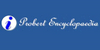### SubtangentSub·tan'gent noun (Geom.) The part of the axis contained between the ordinate and tangent drawn to the same point in a curve.
Found on http://www.encyclo.co.uk/webster/S/228

### Subtangent• (n.) The part of the axis contained between the ordinate and tangent drawn to the same point in a curve.
Found on http://thinkexist.com/dictionary/meaning/subtangent/

### SubtangentA subtangent is the segment of a produced axis intercepted between an ordinate and a tangent, both drawn from the same point in the curve.
Found on http://www.probertencyclopaedia.com/browse/GS.HTM

### SubtangentIn geometry, the subtangent and related terms are certain line segments defined using the line tangent to a curve at a given point and the coordinate axes. The terms are somewhat archaic today but were in common use until the early part of the 20th century. ==Definitions== Let P = (x, y) be a point on a given curve with A = (x, 0) its projection o...
Found on http://en.wikipedia.org/wiki/Subtangent
No exact match found